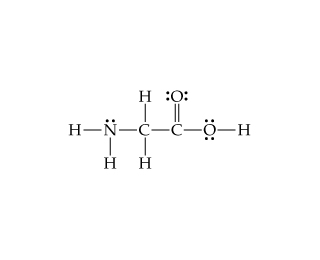Chemistry Practice Problems Bond Angles Practice Problems Solution: Consider the Lewis structure for glycine, the simp...

🤓 Based on our data, we think this question is relevant for Professor Dixon's class at UCF.

# Solution: Consider the Lewis structure for glycine, the simplest amino acid: What are the approximate bond angles at the nitrogen?

###### Problem

Consider the Lewis structure for glycine, the simplest amino acid:What are the approximate bond angles at the nitrogen?

###### Solution

We’re being asked to identify the bond angles around nitrogen in glycineSince the Lewis structure for glycine is already given, we simply need to do the following steps:

Step 1: Determine the number of electron groups around the indicated atom.

Step 2: Determine the electron geometry and bond angle using this:

Electron Regions          Electronic Geometry          Bond Angles

2                                      linear                                    180˚

3                                      trigonal planar                      120˚

4                                      tetrahedral                            109.5˚

5                                      trigonal bipyramidal              90˚, 120˚, and 180˚

6                                      octahedral                            90˚ and 180˚View Complete Written Solution

Bond Angles

Bond Angles

#### Q. The compound with the following Lewis structure is acetylsalicylic acid, better known as aspirin: .What are the approximate values of the bond angles ...

Solved • Mon Nov 12 2018 15:48:57 GMT-0500 (EST)

Bond Angles

#### Q. In a trigonal-bipyramidal geometry, there are two types of positions for the outer atoms.What is the bond angle formed by an axial atom, the central a...

Solved • Mon Nov 12 2018 15:48:55 GMT-0500 (EST)

Bond Angles

#### Q. Use VSEPR to predict the geometry (including bond angles) about each interior atom of methyl azide (CH3N3), and make a sketch of the molecule. Would y...

Solved • Mon Nov 12 2018 12:41:36 GMT-0500 (EST)

Bond Angles

#### Q. Suppose that a molecule has four bonding groups and one lone pair on the central atom. Suppose further that the molecule is confined to two dimensions...

Solved • Mon Nov 12 2018 12:41:11 GMT-0500 (EST)